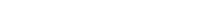# Math riddles Level 37 Answer with Hints and solutionsMath riddles game level 37 Answer with solution android game developed by Black games. Scroll Below to find details.

Math Riddles tests your IQ with mathematical puzzles. Challenge yourself with different levels of math puzzles and stretch the limits of your intelligence. Every IQ game is prepared with an approach of an IQ test. Improve your mathematics, challenge your brain with this game. There are many hard levels which you may not have solved then you can take help from here.

Math app level 37:

3,6=27

5,2=17

4,1=9

2,7=?Solution:

3*6+(3+6)=27

5*2+(5+2)=17

similarly

2*7+(2+7)=23

If there is any Doubt/discrepancy then please let me know in comments or you are unable to understand solution then I will surely help you.

### 4 thoughts on “Math Riddles App Level 37 Solution Android”

•September 26, 2019 at 3:44 am

thanks thanks!!

•August 13, 2019 at 2:39 pm

3,6=27

5,2=17

4,1=9

2,7=?

(3*6)+4+5=27
(5*2)+3+4=17
(4*1)+2+3=9
(2*7)+3+4+5+6=32

•July 27, 2019 at 4:42 am

In my game it shows the following:

3,6=13
5,2=8
4,1=9
2,7=?

No wonder I couldn’t solve it. Guess I’m done with this app.

•